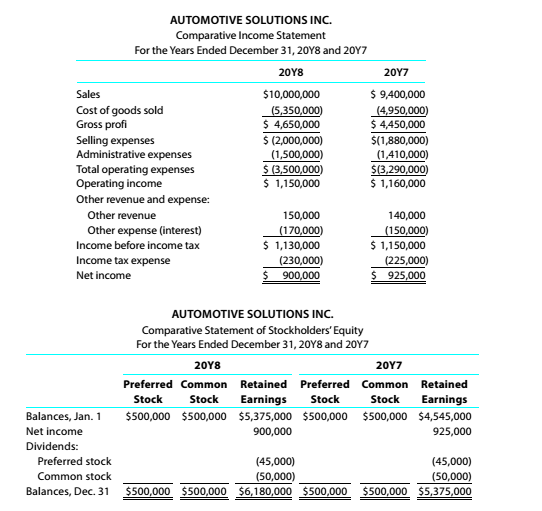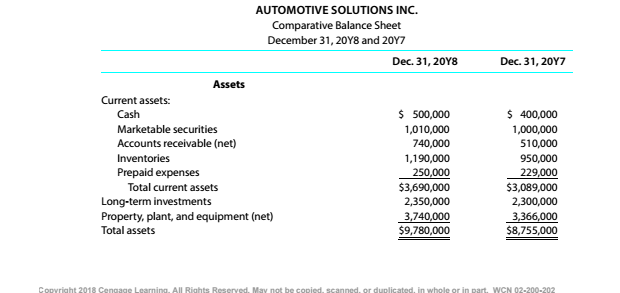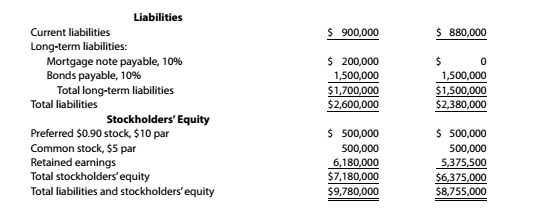Chapter 9, Problem 9.4.3P

Chapter
Section
Textbook Problem

Twenty metrics of liquidity, solvency, and profitabilityThe comparative financial statements of Automotive Solutions Inc. are as follows. The market price of Automotive Solutions Inc. common stock was $119.70 on December 31, 20Y8InstructionsQuick ratio To determine Concept Introduction: Acid test ratio: Acid test ratio is also called Quick ratio. This ratio is calculated by dividing the quick assets (Cash, Cash equivalents, Short term investments and current receivables) by total current liabilities for the year. The formula for Acid test ratio is as follows: Acid test ratio = (Cash + Cash equivalents + Short term investments + Accounts receivables) Current Liabilities To Calculate: The Quick Ratio. Explanation The Quick Ratio is calculated as follows:  20Y8 20Y7 Total Current Assets$ 3,690,000 $3,089,000 Less: Inventories$ (1,190,000) \$ (950,000)

Still sussing out bartleby?

Check out a sample textbook solution.

See a sample solution

The Solution to Your Study Problems

Bartleby provides explanations to thousands of textbook problems written by our experts, many with advanced degrees!

Get Started

What does the invisible hand of the marketplace do?

Essentials of Economics (MindTap Course List)

To put XOMs P/E ratio in perspective, it is useful to see how this ratio has varied over time. (If you go to Mo...

Fundamentals of Financial Management, Concise Edition (with Thomson ONE - Business School Edition, 1 term (6 months) Printed Access Card) (MindTap Course List)

How do unions affect the natural rate of unemployment?

Principles of Macroeconomics (MindTap Course List)